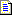English (English)

# Hypotheses for Static Text elements

When the program looks for static text on an image, the recognized text need not exactly match the text specified in the properties of the element.

For example, you can specify the properties of a Static Text element in such a way as to make the program consider the object "Supplier" as a valid hypothesis for the expected static text "Supplier".

You can set the following parameters in the Element Properties dialog box:

• Max number of errors – the maximum number of incorrect characters in the word
• Max error percentage – the percentage of errors allowed in the word (calculated as a ratio of the number of errors in the hypothesis to the total number of characters in the hypotheses)

If the number of errors exceeds the set threshold, the detected object will not be considered a valid hypothesis. The greater the number of errors, the poorer the quality of the hypothesis. Since the number of characters in the object is not known in advance and depends on what images are processed, the program will use either the threshold set in absolute numbers or the threshold set in percentage points. When formulating and evaluating a hypothesis, the program will use the more stringent of the two conditions, i.e. if the detected object meets one criterion but does not meet the other criterion, the object will not be considered a valid hypothesis.

A Static Text hypothesis has the following properties:

 Property Description Element name The full name of the element. Page The number of the page on which the element was detected. Surrounding rect The coordinates of the rectangle which surrounds the region of the hypothesis. Width The width of the region of the hypothesis. Height The height of the region of the hypothesis. Keyword Lists the found keywords which you set in the Search text field. For each word, the program shows the number of errors. Detected Shows whether the object described by the element has been found (true) or whether a null hypothesis has been formulated (false). From the best path Shows whether the found hypothesis belongs to the best path in the tree of hypotheses (true) or not (false). Pre-search quality How well the hypothesis matches the properties of the element specified by the settings in the Properties dialog box and by the code in the Advanced pre-search relations field. Post-search quality The quality of the hypothesis after the conditions in the Advanced post-search relations field have been applied. Chain quality The quality of the chain of hypotheses, from the first subelement of the group to the current subelement. Chain quality is calculated by multiplying the qualities of all the subelements in the chain and is used to compare rival chains of hypotheses.

#### More:Static TextSearch areaAdditional search constraints

25.05.2023 7:55:03